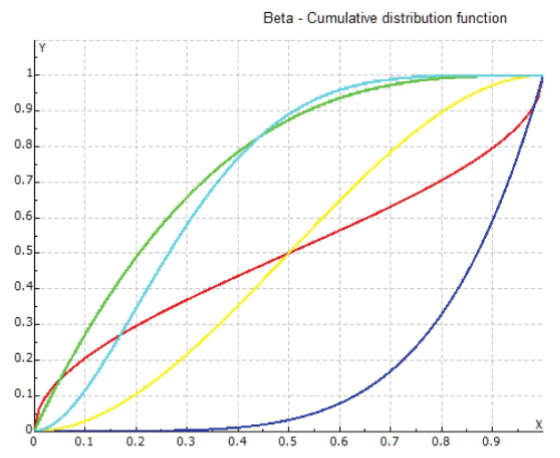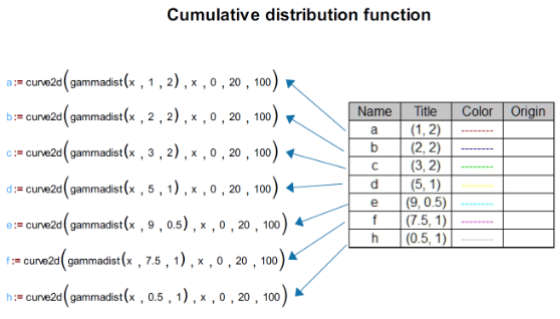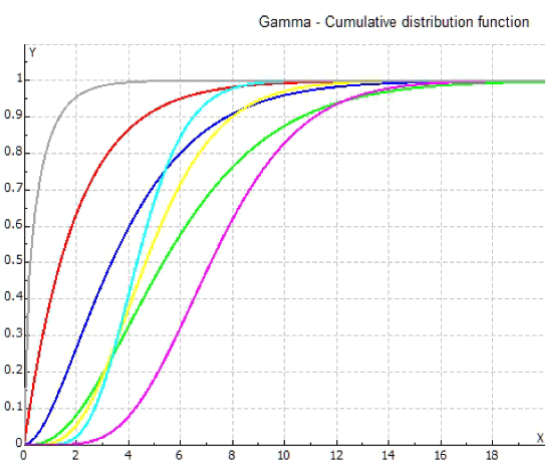# Probability Distributions

A probability distribution is a mathematical function that provides the probabilities of different possible outcomes in an example occurring. It tells you what the probability of a certain event happening.

In MatDeck, for each distribution option that is provided in the software we have included a Cumulative Distribution Function (CDF), Probability Distribution Function (PDF) and Inverse Cumulative Distribution Function.

PDF’s are widely used in actuarial science, engineering, physics, computer science, evolutionary biology, psychiatry, economics, medical trials… They are essential in many applied fields of science and modern day life.

CDF’s are more suitable for modeling real-world problems which have sudden changes in adjacent variables.The Probability Density Functions and Cumulative Distribution Functions in MatDeck are:

• betadens – PDF Beta distribution
• betadist – CDF Beta distribution
• betainv – Inverse CDF Beta distribution
• cauchydens – PDF Cauchy distribution
• cauchydist – CDF Cauchy distribution
• cauchyinv – Inverse CDF Cauchy distribution
• chidens – PDF Chi distribution
• chidist – CDF Chi distribution
• chiinv – Inverse CDF Chi distribution
• dblexponentialdens – PDF Double Exponential distribution
• dblexponentialdist – CDF Double Exponential distribution
• dblexponentialinv – Inverse CDF Double Exponential distribution
• exponentialdens – PDF Exponential distribution
• exponentialdist – CDF Exponential distribution
• exponentialinv – Inverse CDF Exponential distribution
• fdens – PDF F-distribution
• fdist – CDF F-distribution
• finv – Inverse CDF F-distribution
• gammadens – PDF Gamma distribution
• gammadist – CDF Gamma distribution
• gammainv – Inverse CDF Gamma distribution
• geometricdens – PDF geometric distribution
• geometricdist – CDF geometric distribution
• geometricinv – Inverse CDF geometric distribution
• logarithmicdens – PDF Logarithmic distribution
• logarithmicdist – CDF Logarithmic distribution
• logarithmicinv – Inverse CDF Logarithmic distribution
• normaldens – PDF Normal (Gaussian) distribution
• normaldist – CDF Normal (Gaussian) distribution
• normalinv – Inverse CDF Normal (Gaussian) distribution
• poissondens – PDF Poisson distribution
• poissondist – CDF Poisson distribution
• poissoninv – Inverse CDF Poisson distribution
• tdens – PDF Student’s t-distribution
• tdist – CDF Student’s t-distribution
• tinv – Inverse CDF Student’s t-distributionBeside these functions, there is a set of Random Number Generator functions which generate random numbers from various different distributions. There are three functions for every supported distribution; they each differ in the form the calculated result is in. You can generate them as a real number, a vector of real numbers or a matrix of real numbers.

Random Number Generators are:

• betarandnum – Random number created with Beta distribution
• betarandvec – Vector of random numbers created with Beta distribution
• betarandmat – Matrix of random numbers created with Beta distribution
• cauchyrandnum – Random number created with Cauchy distribution
• cauchyrandvec – Vector of random numbers created with Cauchy distribution
• cauchyrandmat – Matrix of random numbers created with Cauchy distribution
• chi2randnum – Random number created with Chi square distribution
• chi2randvec – Vector of random numbers created with Chi square distribution
• chi2randmat – Matrix of random numbers created with Chi square distribution
• dblexprandnum – Random number created with Double Exponential distribution
• dblexprandvec – Vector of random numbers created with Double Exponential distribution
• dblexprandmat – Matrix of random numbers created with Double Exponential distribution
• exprandnum – Random number created with Exponential distribution